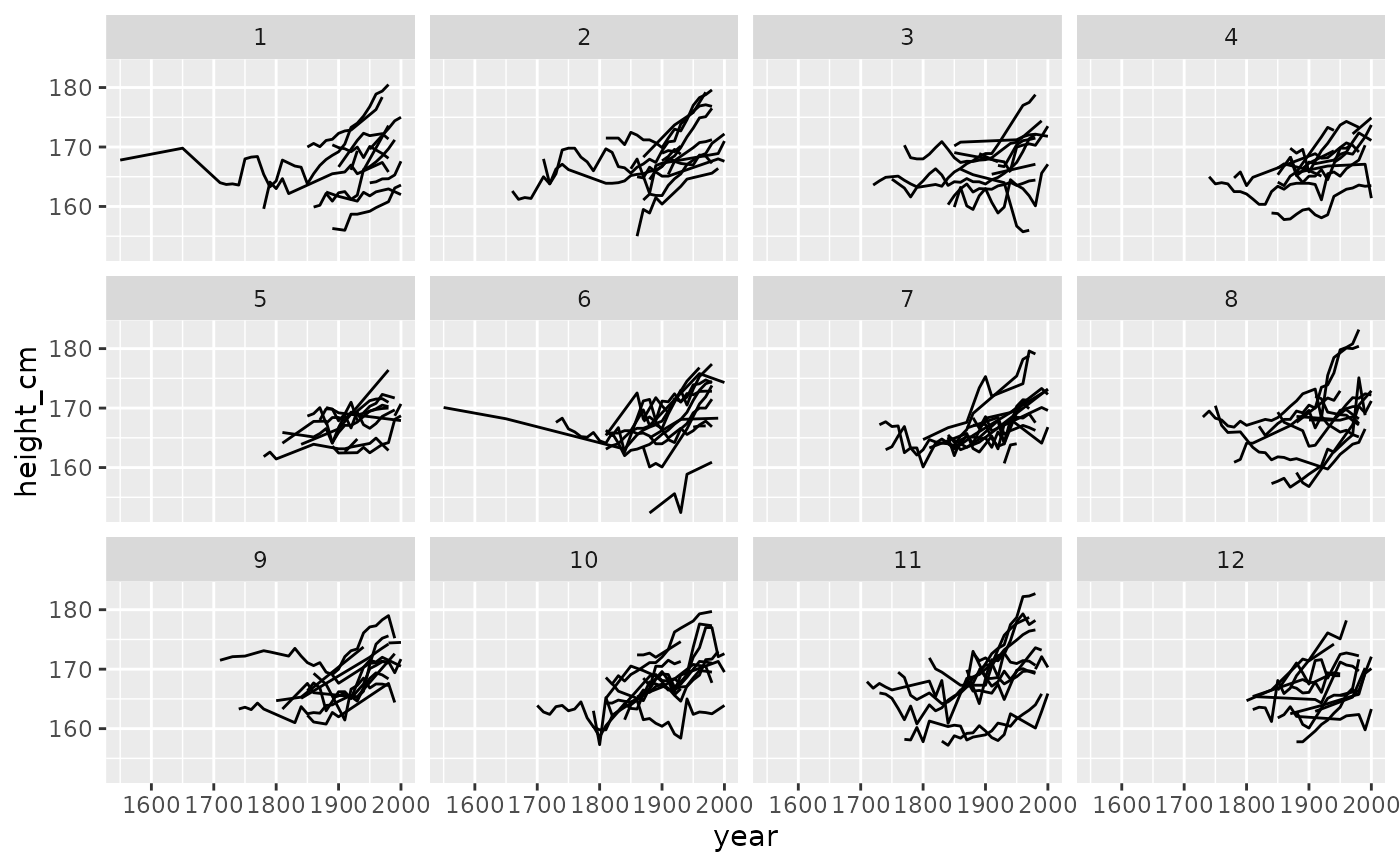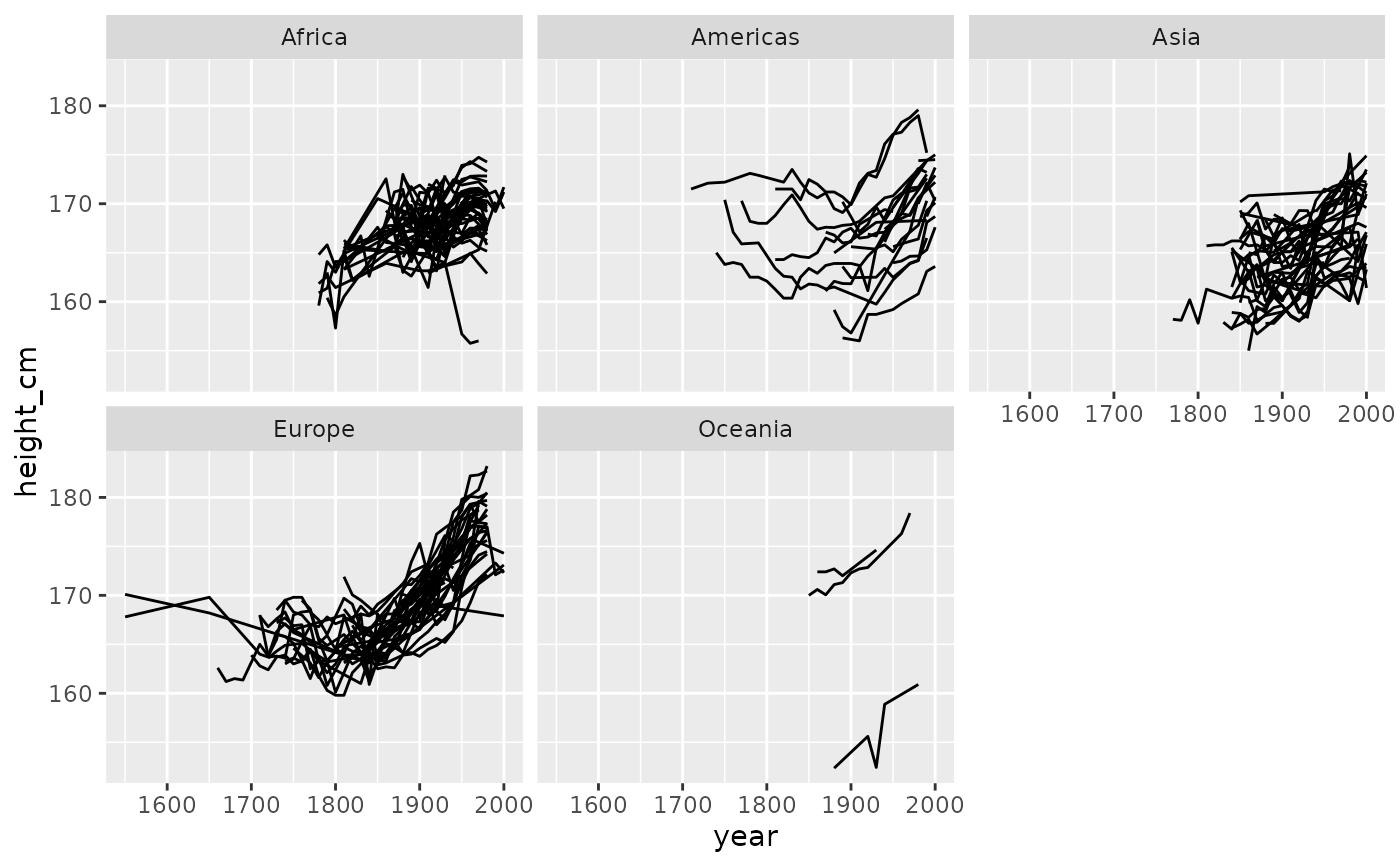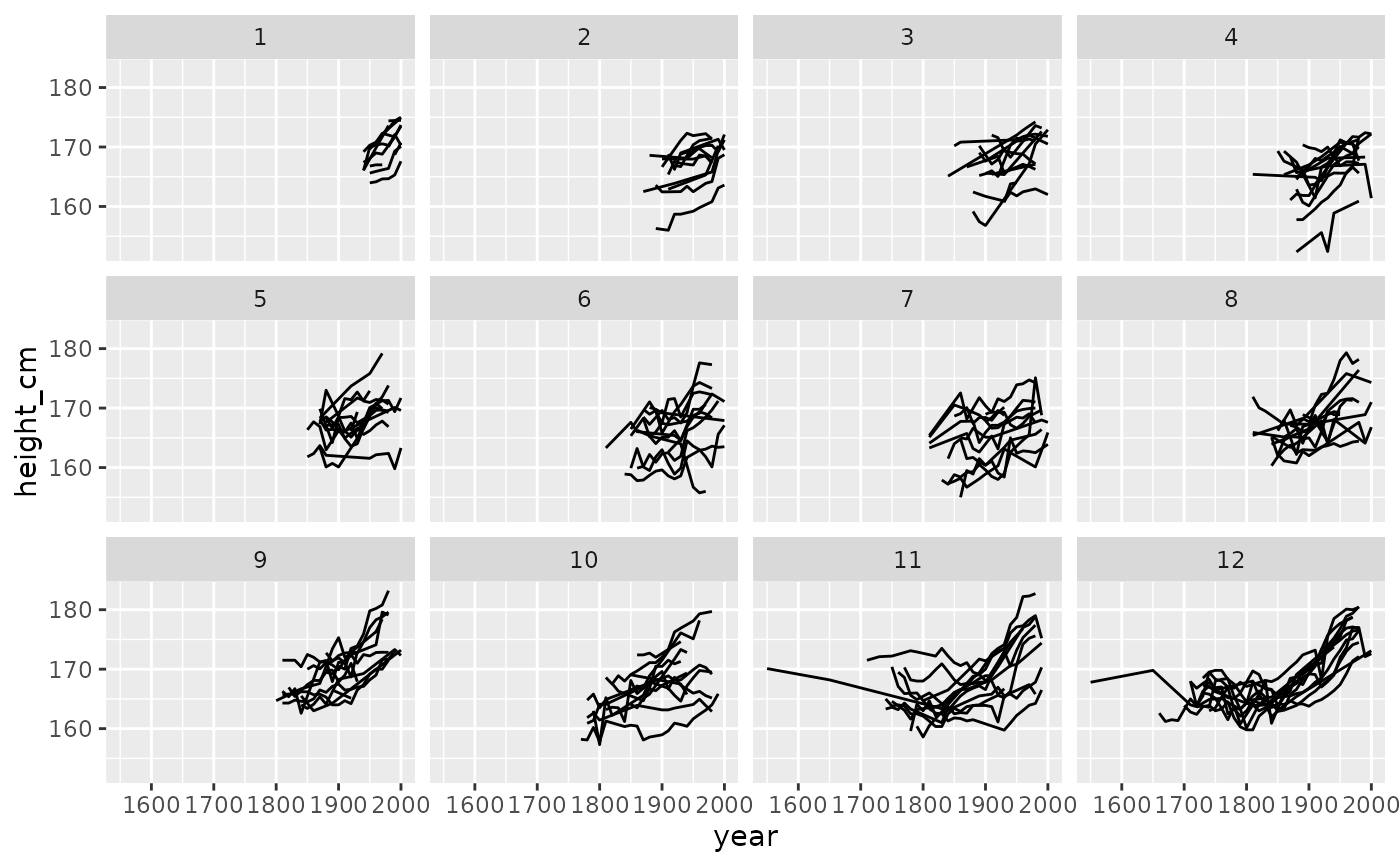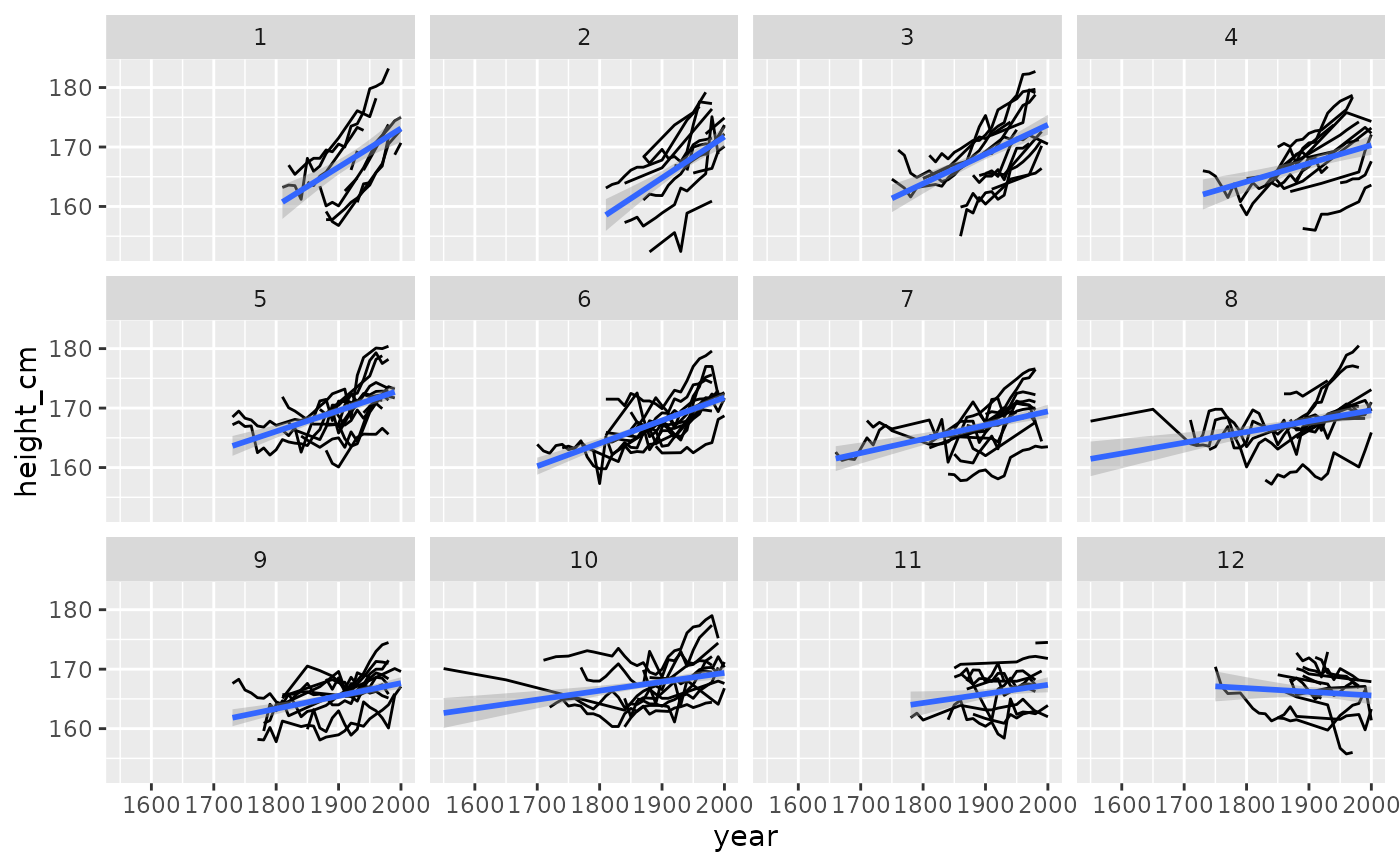This function requires a tbl_ts object, which can be created with tsibble::as_tsibble(). Under the hood, facet_strata is powered by stratify_keys().

## Usage

facet_strata(
n_strata = 12,
along = NULL,
fun = mean,
nrow = NULL,
ncol = NULL,
scales = "fixed",
shrink = TRUE,
strip.position = "top"
)

## Arguments

n_strata

number of groups to create

along

variable to stratify along. This groups by each key and then takes a summary statistic (by default, the mean). It then arranges by the mean value for each key and assigns the n_strata groups.

fun

summary function. Default is mean.

nrow, ncol

Number of rows and columns.

scales

Should scales be fixed ("fixed", the default), free ("free"), or free in one dimension ("free_x", "free_y")?

shrink

If TRUE, will shrink scales to fit output of statistics, not raw data. If FALSE, will be range of raw data before statistical summary.

strip.position

By default, the labels are displayed on the top of the plot. Using strip.position it is possible to place the labels on either of the four sides by setting strip.position = c("top", "bottom", "left", "right")

a ggplot object

## Examples

library(ggplot2)
ggplot(heights,
aes(x = year,
y = height_cm,
group = country)) +
geom_line() +
facet_strata()ggplot(heights,
aes(x = year,
y = height_cm,
group = country)) +
geom_line() +
facet_wrap(~continent)ggplot(heights,
aes(x = year,
y = height_cm,
group = country)) +
geom_line() +
facet_strata(along = year)# \donttest{
library(dplyr)
heights %>%
key_slope(height_cm ~ year) %>%
right_join(heights, ., by = "country") %>%
ggplot(aes(x = year,
y = height_cm)) +
geom_line(aes(group = country)) +
geom_smooth(method = "lm") +
facet_strata(along = .slope_year)
#> geom_smooth() using formula = 'y ~ x'# }